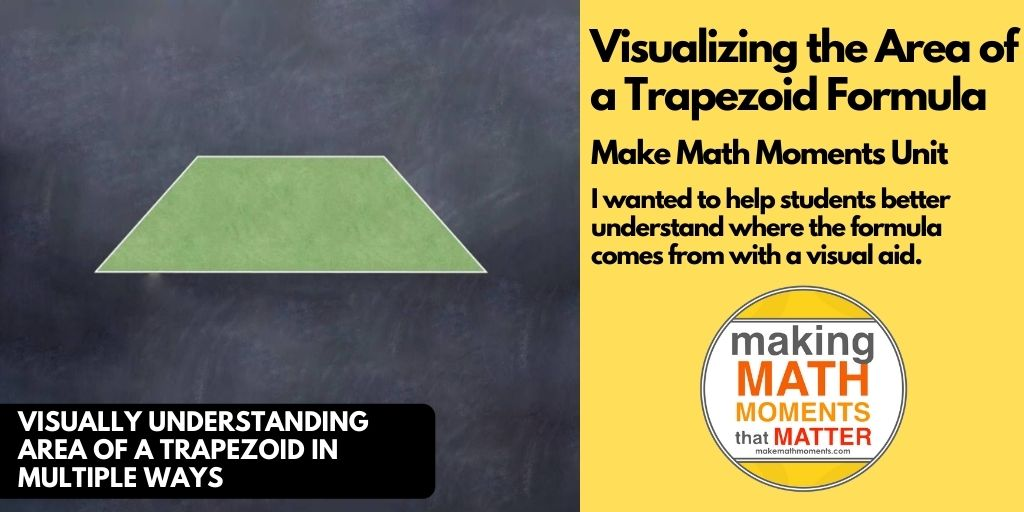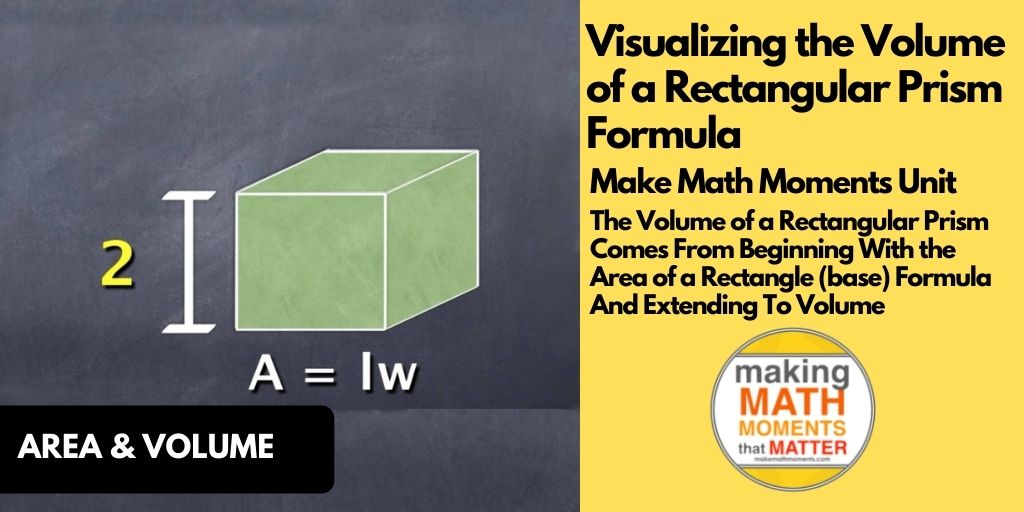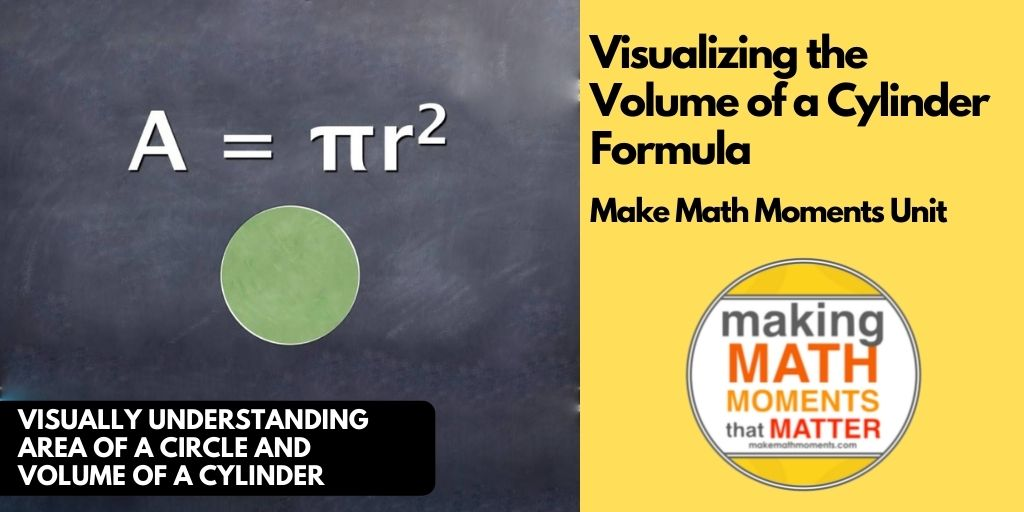## Visualizing the Area of a Trapezoid Formula

Visually Understanding Area of a Trapezoid in Multiple Ways While some students are aware that they could find the area of a trapezoid by treating the shape like a composite figure, I wanted to help students better understand where the formula comes from with a visual...## Visualizing the Volume of a Rectangular Prism Formula

Visually Understanding Area of a Rectangle and Volume of a Rectangular Prism Yesterday, I showed the “Where Does the Volume of a Cylinder Formula Come From?” video in class and students seemed to be able to tie some of the visuals to the work they...## Visualizing the Volume of a Cylinder Formula

Visually Understanding Area of a Circle and Volume of a Cylinder Over the past year, I have been on a mission to try and make some of the formulas we use in the intermediate math courses in Ontario (Middle School for our friends in the U.S.). I think it can be...